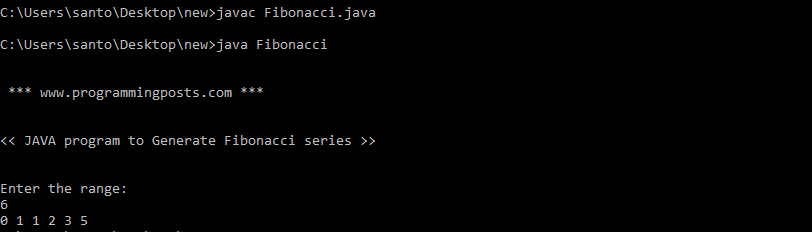# Java program to Generate Fibonacci sequence

A series of numbers in which each number is the sum of the two preceding numbers.

for example 0, 1, 1, 2, 3, 5, 8, 13, 21, 34, 55 etc. The first two numbers of fibonacci series are 0 and 1.

### Algorithm:

Step 1: START.

Step 2: Declare variables a,b,c.

Step 3: Initialize the variables.

Step 4: Enter no of terms of terms of fibonacci series to be printed.

Step 5: Print a,b.

Step 6: Use loop for the following steps
c=a+b
a=b
b=c

Step 7: Print c.

Step 8: STOP.

### Source code:

```import java.util.*;
class Fibonacci
{
public static void main(String args[])
{
int a=0;
int b=1;
int c=0;
System.out.println("\n \n *** www.programmingposts.com *** \n \n");
System.out.println("<< JAVA program to Generate Fibonacci series >> \n\n ");
System.out.println("Enter the range:");
Scanner scanner = new Scanner(System.in);
int range=scanner.nextInt();
System.out.print(a);
System.out.print(" "+b);
for(int i=2;i<range;i++)
{
c=a+b;
System.out.print(" " +c);
a=b;
b=c;
}
}
}```

### Explanation:

This program calculates the Fibonacci series up to a limit and print the output back to the user.
The program has several variables –
• a, b, c – These integer variables are used for the calculation of Fibonacci series. The values of a, b and c are initialized to 0, 1 and 0 respectively.
• range – This integer is the limit determining the number of elements of Fibonacci series that should be calculated.
• i – This is the loop variable.
First the user enters a limit. After that for loop takes the control and calculates the Fibonacci series up to the previously entered limit.
The loop starts from 2 because 0 and 1 are already printed.
Process for i = 2
• First we update the value of c (initially 0) with the following expression – c = a + b. So after this c = 0+ 1 = 1 (Third value of Fibonacci series).
• Then we print the value of c.
• At last we update the value of a as – “a = b” and then the value of b as – “b = c”. So after this step a = 1 and b = 1.

This process continues till the condition in the loop gets satisfied.

### Output:

C:\Users\santo\Desktop\new>javac Fibonacci.java

C:\Users\santo\Desktop\new>java Fibonacci

*** www.programmingposts.com ***

<< JAVA program to Generate Fibonacci series >>

Enter the range:
6
0 1 1 2 3 5

### Screenshot of Output:Screenshot of output